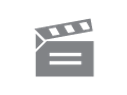Media not available in the Digital Archive
Description
This programme discusses the elements of projective geometry and transformations in the projective plane. These qualities are then used to prove a theorem.
Module code and title: M203, Introduction to pure mathematics M203; 21 17-07-1981 1981 00:21:35 + Show more... David Saunders David Crow; Allan I.,1936-2013 Solomon BBC Open University Perspective Changes; Plane; Projective Geometry Allan Solomon introduces the programme by demonstrating how angles alter their appearance when viewed from different positions. This is perspective and the study of perspective in mathematics is Projective Geometry. He lists properties that are not preserved in projective geometry, angles, lengths, ratio of lengths and circles. Properties preserved are points, straight lines and incidence. David Crowe examines two statements about line and points to see if they hold true under projective geometry. Given any two points there's a unique straight line which passes through both of them. Given any two lines there is a unique point which lies on both of them. Having discussed these statements he expands them to include the case of parallel lines, arguing that they can be thought of as meeting at a point in infinity. These points are called ideal points. He then shows that all the ideal points lie on a straight line, the ideal line. Having discussed the idea of ideal points and lines. David now returns to his two statements to check that they hold true for these new possibilities. Allan Solomon now discusses theorems in projective geometry. He describes a theorem and then constructs the theorem for himself. The theorem states that for any point within a triangle, join each vertex to this point, continuing to the opposite side. These new points make a triangle within the old triangle. Extend the corresponding sides of the triangles until they meet, this gives three points and these points all lie on a straight line. Having shown that this is so, Allan argues that this is a theorem in protective geometry as it only involves points, straight lines and incidence. It should therefore be possible to prove the theorem using the methods of projective geometry. These methods involve transformations of the projective plane. David Crowe describes how these transformations can be represented. Using the example of the image seen through a TV lens he shows how the Domain and of the transformation can be thought of as lying on an object and image plane, respectively. Points or the two planes are related by straight lines, passing through a fixed point. He shows that by choosing angles of the planes and the position of the fixed point, carefully, it is possible to transform a circle to a hyperbola. This is because they are both conic sections. Using a model of the cone he relates points on the object plane to points on the image plane. He demonstrates that for projective planes each point has an image in the image plane The image of some points are ideal points in the image plane. Allan Solomon now uses the representation of projective transformationsto prove his earlier theorem. He tries the theorem against a special case, an equilateral triangle. Using ideal points he shows that the theorem is true. He then moves from the special case to the general and proves this to be true by projecting the equilateral triangle onto a general triangle. DOU3043 FOUM021A 4103 no